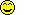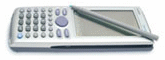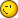# Armonic Funtions

6 replies to this topic

### #1manuelfrank12

manuelfrank12

Casio Fan

•• Members
•• 34 posts

• Calculators:

Posted 30 April 2005 - 09:13 PM

somebody now if the classpad can transform
12 cos(x+45)+ 13 sen(x)

to a funtion like
A cos(x+)

if, it is possible how can i do it?.
manuel franco

### #2Lovecasio

Lovecasio

Casio Freak

•• Members
•• 242 posts
• Location:Hochiminh city Vietnam
• Interests:Organic chemistry.<br />Pharmacy

• Calculators:
fx 570 MS, Casio AFX 2.0+, ClassPad 300

Posted 01 May 2005 - 07:30 AM

Hi.
I'm afraid it cannot do your requirement right now. (I'm sorry but I think it is sin, not sen, isn't it?)
Ex: sin(x+pi/6) = sinx*sqrt(3)/2 + cosx/2 ( this kind CP can do )
But: ask CP to do the inverse, he can't.### #3manuelfrank12

manuelfrank12

Casio Fan

•• Members
•• 34 posts

• Calculators:

Posted 04 May 2005 - 01:23 AM

Hi.
I'm afraid it cannot do your requirement right now. (I'm sorry but I think it is sin, not sen, isn't it?)
Ex: sin(x+pi/6) = sinx*sqrt(3)/2 + cosx/2 ( this kind CP can do )
But: ask CP to do the inverse, he can't.<{POST_SNAPBACK}>

yes, you are rigth is sin not sen
a problen with the languaje

is very bad that the classpad can't do that , look easy to do
thanks

### #4betoe

betoe

UCF Spanish Translator

•• [Legends]
•• 846 posts
• Gender:Male
• Interests:Electronics, SW development, automotive. Swim->bike->run

• Calculators:

Posted 04 May 2005 - 04:59 AM

I think it can but i dont know the exactly command sequence :s.

### #5R00KIE

R00KIE

Casio Freak

•• Members
•• 155 posts
• Location:Portugal
• Interests:Electronics, games, programming

• Calculators:
HP49G ROM 1.24; CASIO CFX-9850GB PLUS;CASIO FX-6300G; CASIO FX-82TL

Posted 08 May 2005 - 05:19 PM

i've tried it in my hp and it did not do it.
i can ask it to rewrite an expression only with sin or cos but result was the same expression, sory i don't think it is possible, and even if you could turn sin into cos i think it would still be very difficult to get to A*cos(x+theta)

### #6Lianna

Lianna

Newbie

•• Members
•• 14 posts

• Calculators:
CFX-9850G

Posted 22 May 2005 - 09:59 PM

somebody now if the classpad can transform
12cos(x+45) + 13sin(x)
to a funtion like
A cos(x+)
if, it is possible how can i do it?.
manuel franco

Define harm(a1,p1,a2,p2)=simplify(sqrt(a1^(2)+a2^(2)+2*a1*a2*cos(p2-p1)))*sin(x+simplify(tan^-1((a1*sin(p1)+a2*sin(p2))/(a1*cos(p1)+a2*cos(p2)))))

Change sqrt to square root symbol and tan^-1 to arctan (arcus tangens) symbol.
If you want to sum cos(x)+sin(x)=1*sin(x+.5*pi)+1*sin(x+0):
harm(1, .5*pi, 1, 0)
In the case of your example that would be:
harm(12, 3*pi/4, 13, 0)
Frequency of input and output must be the same and is set as 1.
Change it to whatever you like.
Best regards,
Lianna

### #7manuelfrank12

manuelfrank12

Casio Fan

•• Members
•• 34 posts

• Calculators:

Posted 23 May 2005 - 11:03 PM

Define harm(a1,p1,a2,p2)=simplify(sqrt(a1^(2)+a2^(2)+2*a1*a2*cos(p2-p1)))*sin(x+simplify(tan^-1((a1*sin(p1)+a2*sin(p2))/(a1*cos(p1)+a2*cos(p2)))))

Change sqrt to square root symbol and tan^-1 to arctan (arcus tangens) symbol.
If you want to sum cos(x)+sin(x)=1*sin(x+.5*pi)+1*sin(x+0):
harm(1, .5*pi, 1, 0)
In the case of your example that would be:
harm(12, 3*pi/4, 13, 0)
Frequency of input and output must be the same and is set as 1.
Change it to whatever you like.
Best regards,
Lianna

<{POST_SNAPBACK}>

that's a very good answer, i didn't think in that way to do itthank
manuel franco

#### 0 user(s) are reading this topic

0 members, 0 guests, 0 anonymous users